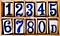# Object-Oriented JavaScript — NumbersPhoto by Diego González on Unsplash

JavaScript is partly an object-oriented language.

To learn JavaScript, we got to learn the object-oriented parts of JavaScript.

In this article, we’ll look at the building blocks of objects, which are primitive values.

# Primitive Data Types

JavaScript has a few primitive data types.

They’re numbers, strings, booleans, `undefined` , `null` , and bigints.

Numbers are floating-point numbers and integers.

Strings are any group of characters.

Booleans are either `true` or `false` .

`undefined` is a value that doesn’t exist.

`null` represents an empty value.

Bigints are integers that end with an `n` and can be outside of the safe range, which is between `-2 ** 53` and `2 ** 53` .

Any value that isn’t these types are objects.

# Finding Out the Value Type

We can find the value of a primitive value with the `typeof` operator.

`typeof` can return `'number'` . `'string'` , `'boolean'` , `'undefined'` , `'object'` or `'function'` .

Numbers are one of the types that can be detected with `typeof` .

For instance, we can write:

`let n = 1;typeof n;`

and we’ll get `'number'` .

Octal and hexadecimal numbers also returns `'number'` .

For instance, we can write:

`let n = 0o377;typeof n;`

to write an octal number and check its type.

To check a hex number, we can write:

`let n = 0x00;typeof n;`

That will also return `'number'` .

We can also write binary literals with the `0b` prefix,.

For instance, we can write:

`let n = 0b111;`

Exponents can be written with `e` .

For instance, we can write:

`1e1`

and get 10.

If we pass it to `typeof` , we get `'number'` :

`typeof 1e1`

Infinity is another kind of number.

It’s a number too big for JavaScript to handle.

`Infinity` is a number, so if we write:

`typeof Infinity`

we get `'number'` .

Dividing by 0 gives us infinity. For instance, if we write:

`let a = 1 / 0`

then `a` is `Infinity` .

The smallest number is `-Infinity` .

When we have:

`Infinity - Infinity`

or

`- Infinity + Infinity`

we get `NaN` since they are indeterminate in their value.

But everything else gives us `Infinity` or `-Infinity` .

For instance, we can write:

`Infinity - 20`

we get `Infinity` .

If we write:

`-Infinity * 3`

we get `-Infinity` .

There’s a global `isFinite` function to check is a number is finite or not.

ES6 also adds the `Number.isFinite` to do the same check.

The difference is that the global `isFinite` function casts the value before it does the check.

And `Number.isFinite` doesn’t do that.

`NaN` stands for not a number.

It’s a special value that’s also a number.

If we write:

`typeof NaN`

we get `'number'` .

When we do some arithmetic with non-number values, then we get `NaN` .

For example, if we have:

`let a = 10 * "a"`

We get `NaN` .

We can check if a value is `NaN` with the `Number.isNaN` method.

There’s also the global `isNaN` method.

The difference is that the global one does casting and the non-global one doesn’t.

So:

`Number.isNaN('test')`

returns `false` but

`Number.isNaN(NaN)`

returns `true` .

`Number.isInteger` is a method that checks if a value is a finite integer.

For instance, if we have:

`Number.isInteger(123)`

then that returns `true` .

But if we have:

`Number.isInteger('foo')`

that returns `false` .

It doesn’t do casting before it does the comparison.Photo by Andrew Buchanan on Unsplash

# Conclusion

There are various kinds of primitive values in JavaScript.

One of them is a number.

There’re various representations of numbers.

Web developer. Subscribe to my email list now at https://thewebdev.info/subscribe/. Email me at hohanga@gmail.com

Web developer. Subscribe to my email list now at https://thewebdev.info/subscribe/. Email me at hohanga@gmail.com